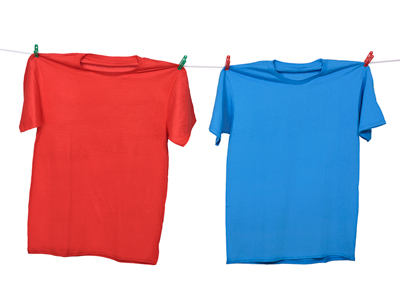Melanie has 18 red tops. How many blue tops does she have?

# Ratio 3 (Medium)

You have made it to the third quiz in our Medium level Eleven Plus maths quizzes on Ratios. Give yourself a pat on the back! If you have already played the first two quizzes, then you will know that ratios are one way of comparing different proportions. They are very similar to fractions, but not exactly the same.

Imagine that you have 4 oranges and 6 apples. You can represent this as a fraction like so: 25 of the fruit are oranges, and 35 are apples.

You can represent the same information as a ratio like this: the ratio of oranges to apples is 2:3. Can you see the similarity? 25 and 35 = 2:5

Now for the quiz. Make sure you read the questions carefully – there’s at least one in there which might catch out the unwary!

1.
63 meals are to be served at a wedding party. If the ratio of vegetarian meals to non-vegetarian meals is 2:5, how many vegetarian meals will be served?
14
16
18
20
2 + 5 = 7 ∴ the fractional parts are 27 x 63 = 18 vegetarian meals, and 57 x 63 = 45 non-vegetarian meals.
Check that 18:45 = 2:5 by dividing by 9. Your answer MUST preserve (keep) the same ratio
2.
1624 of the patients at a vet’s are cats and the rest are dogs. What is the simplest way of representing this as a ratio of dogs to cats?
2:1
8:16
1:2
16:8
If 1624 of the animals are cats, then 824 are dogs (24 - 16 = 8)
These can be simplified to 23 cats and 13 dogs by dividing by 8 (16 ÷ 8 = 2, and 8 ÷ 8 = 1)
So, the ratio of cats to dogs is 2:1, BUT we were asked for the ratio of dogs to cats, so we must reverse the ratio to 1:2
Watch out for tricks like this!
3.
The ratio of bean cans to soup cans in a shop is 2:7. What fraction of the cans contain soup?
27
57
29
79
2 + 7 = 9 so the fraction will be in ninths. You DON'T have to know what the total number of items is in order to find the fractional amounts of each type of item
4.
Which of the following is not a way of expressing the ratio 2:7?
6:21
14:4
10:35
8:28
The ratio 14:4 = 7:2 which is NOT the same as 2:7. All the others form ratios that can be reduced to 2:7
5.
Melanie has 18 red tops. How many blue tops does she have if the ratio of red tops to blue tops is 6:5?
11
13
15
17
For every 6 red tops, Melanie has 5 blue ones.
18 ÷ 6 = 3
3 x 5 = 15
Melanie has 15 blue tops
6.
How else can the ratio 1.5:8 be written?
2:6
2:16
3:16
3:24
1.5:8 = 3:16 (multiplying by 2)
7.
If the ratio of wind instruments to string instruments in a band is 7:8, which of the following statements is correct?
The number of wind instruments is less than the number of string instruments
The number of wind instruments equals the number of string instruments
The number of wind instruments is more than the number of string instruments
There is not enough information to answer the question
8 is greater than 7 and it represents the number of string instruments. There are clearly more string instruments than wind instruments
8.
The following ratios show the number of fiction to non-fiction books in four bookshops. If each shop has the same number of books in it, which has the largest number of non-fiction books?
5:1
17:14
28:28
10:11
28:28 represents an equal amount of fiction and non-fiction. It could also be shown as 1:1
17:14 and 5:1 both represent a higher number of fiction books, so they are wrong.
10:11 is the only ratio which represents more non-fiction than fiction, so it must be the right answer
9.
The ratio of bananas to apples on a fruit stall is 13:9. If there are 45 apples on the stall, how many bananas are there?
55
65
75
85
45 ÷ 9 = 5, so we must multiply 13 by 5 to find the number of bananas.
5 x 13 = 65
10.
How else can the ratio 14:35 be written?
2:5
2:7
2:9
7:2
14:35 = 2:5 (dividing by 7)
Author:  Frank Evans